>

#### Study Material and Notes of Ch 13 Magnetic Effects of Electric Current Class 10th Science

Topics in the Chapter

• Introduction
• Properties of magnet
→ Characteristics of Field lines
→ Magnetic Field of a Bar Magnet
• Right Hand Thumb Rule
• Magnetic Field due to current through a Straight conductor
• Magnetic field due to Current through a Circular Loop
→ Factors affecting magnetic field of a circular current carrying conductor
• Solenoid
→ Directionof magnetic field
• Electromagnet
• Permanent Magnet
• Force on a current carrying conductor in a Magnetic field
• Fleming's Left Hand Rule
→ MRI (Magnetic Resonance imaging)
→ Galvanometer
• Electric Motor and its working
→ Commutator
→ Armature
→ Commercial use of electric motors
• Electro magnetic Induction
• Fleming's Right Hand Rule
• Electric Generator and its working
• Alternate Current (A.C.)
• Direct current (D.C.)
• Domestic Electric Circuits
→ Earth wire
→ Short circuit
→ Safety devices

Introduction

→ Magnet is any substance that attracts iron or iron-like substances.

→ An electric current-carrying wire behaves like a magnet.

→ Electromagnets and electric motors involve the magnetic effect of electric current, and electric generators involve the electric effect of moving magnets.

→ Compass needle get deflected on passing an electric current through a metallic conductor.Properties of Magnet

(i) Every magnet has two poles i.e. North and South.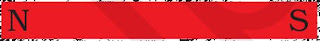(ii) Like poles repel each other.

(iii) Unlike poles attract each other.

(iv) A freely suspended bar magnet aligns itself in nearly north-south direction, with its north pole towards north direction.

Characteristics of Field Lines→ Field lines arise from North pole and end into South pole of the magnet.

→ Field lines are closed curves.

→ Field lines are closer in stronger magnetic field.

→ Field lines never intersect each other as for two lines to intersect, there must be two north directions at a point, which is not possible.

→ Direction of field lines inside a magnet is from South to North.

→ The relative strength of magnetic field is shown by degree of closeness of field
lines.

Magnetic Field of a Bar Magnet

→ H. C. Oersted was the first person to state that electric current has magnetic field.Right Hand Thumb Rule→ Imagine you are holding a current carrying straight conductor in your right hand such
that the thumb is pointing towards the direction of current.

→ Then the fingers wrapped around the conductor give the direction of magnetic field.

Magnetic Field due to Current through a Straight Conductor

→ It can be represented by concentric circles at every point on conductor.

→ Direction can be given by right hand thumb rule or compass.

→ Circles are closer near the conductor.

→ Magnetic field ∝ Strength of current.

→ Magnetic field ∝ 1/Distance from conductor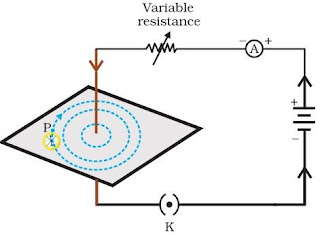Magnetic Field due to Current through a Circular Loop

→ It can be represented by concentric circle at every point.

→ Circles become larger and larger as we move away.

→ Every point on wire carrying current would give rise to magnetic field appearing as straight line at centre of the loop.

→ The direction of magnetic field inside the loop is same.Factors affecting magnetic field of a circular current carrying conductor

→ Magnetic field ∝ Current passing through the conductor

→ Magnetic ∝ 1/Distance from conductor

→ Magnetic field ∝ No. of turns in the coil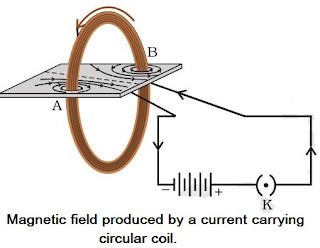→ Magnetic field is additive in nature i.e., magnetic field of one loop adds up to magnetic field of another loop. This is because the current in each circular turn has some direction.

Solenoid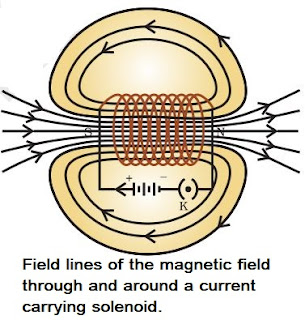→ A coil of many circular turns of insulated copper wire wrapped closely in a cylindrical form.

→ Magnetic field of a solenoid is similar to that of a bar magnet.

→ Magnetic field is uniform inside the solenoid and represented by parallel field lines.

Direction of magnetic field(i) Outside the solenoid: North to South
(ii) Inside the solenoid: South to North

→ Solenoid can be used to magnetise a magnetic material like soft iron.

Electromagnet

→ It is a temporary magnet, so, can be easily demagnetised.

→ Strength can be varied.

→ Polarity can be reversed.

→ Generally strong magnet.

Permanent Magnet

→ Cannot be easily demagnetised.

→ Strength is fixed.

→ Polarity cannot be reversed.

→ Generally weak magnet.

Force on a Current carrying Conductor in a Magnetic Field

Andre Marie Ampere suggested that the magnet also exerts an equal and opposite force
on a current carrying conductor.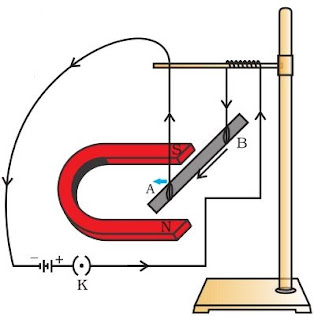→ The displacement in the conductor is the maximum when the direction of current is at right angle to the direction of magnetic field.

→ Direction of force is reversed on reversing the direction of current.

Fleming’s Left Hand Rule

→ Stretch the thumb, fore finger and middle finger of your left hand such that they are mutually perpendicular.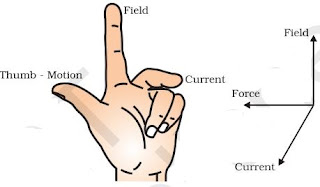→ If fore finger points in the direction of magnetic field, middle finger in the direction of current then thumb will point in the direction of motion or force.

→ Heart and brain in the human body have significant magnetic field.

• MRI (Magnetic Resonance Imaging): Image of internal organs of body can be obtained using magnetic field of the organ.

• Galvanometer: Instrument that can detect the presence of current in a circuit. It also detects the direction of current.

Electric motor

→ An electric motor is a rotating device that converts electrical energy to mechanical energy.→ An electric motor consists of a rectangular coil ABCD of insulated copper wire. The coil is placed between the two poles of a magnetic field such that the arm AB and CD are perpendicular to the direction of the magnetic field.

→ The ends of the coil are connected to the two halves P and Q of a split ring. The inner sides of these halves are insulated and attached to an axle.

→ The external conducting edges of P and Q touch two conducting stationary brushes X and Y, respectively.

→ Current in the coil ABCD enters from the source battery through conducting brush X and flows back to the battery through brush Y.

→ The force acting on arm AB pushes it downwards while the force acting on arm CD pushes it upwards.

→ Thus the coil and the axle O, mounted free to turn about an axis, rotate anti-clockwise.

→ At half rotation, Q makes contact with the brush X and P with brush Y. Therefore the current in the coil gets reversed and flows along the path DCBA.

→ The split ring acts as a commutator which reverse the direction of current and also reverses the direction of force acting on the two arms AB and CD.

→ Thus the arm AB of the coil that was earlier pushed down is now pushed up and the arm CD previously pushed up is now pushed down.

→ Therefore the coil and the axle rotate half a turn more in the same direction. The reversing of the current is repeated at each half rotation, giving rise to a continuous rotation of the coil and to the axle.

• Commutator: A device that reverses the direction of flow of current through a circuit is called a commutator.

• Armature: The soft iron core, on which the coil is wound including the coils is called armature. It enhances the power of the motor.

Commercial use of motors

(i) an electromagnet in place of permanent magnet

(ii) large number of turns of the conducting wire in the current-carrying coil

(iii) a soft iron core on which the coil is wound.

Electro Magnetic Induction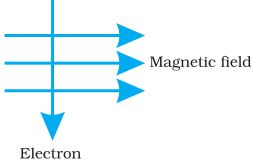→ When a conductor is placed in a changing magnetic field, some current is induced in it.

→ Such current is called induced current and the phenomenon is called electromagnetic induction.

Activity No. 1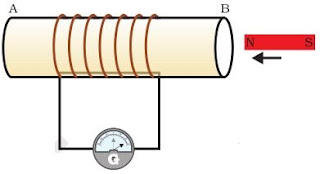(i) Magnet moved into the coil: Momentary deflection in G indicating presence of current.

(ii) Magnet kept stationary inside the coil: No deflection.

(iii) Magnet is withdrawn: Momentary deflection in G but in opposite direction of first case.

Activity No. 2(i) Switched on: Momentary deflection in G.

(iii) Switched off: Momentary deflection in G but in opposite direction of the first case.

Fleming’s Right Hand Rule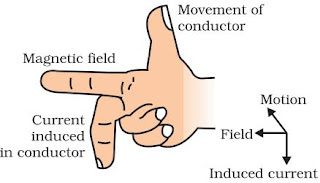→ Hold the thumb, the fore finger and the middle finger of right hand at right angles to each other.

→ If the fore finger is in the direction of magnetic field and the thumb points in the direction of motion of conductor, then the direction of induced current is indicated by middle finger.

• Working principle of electric generator.
• Used to find direction of induced current.

Electric Generator

→ An electric generator, mechanical energy is used to rotate a conductor in a magnetic field to produce electricity.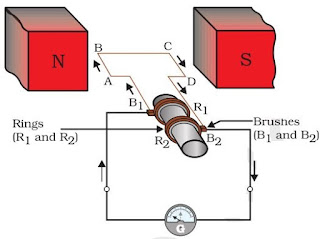→ An electric generator consists of a rotating rectangular coil ABCD placed between the two poles of a permanent magnet.

→ The two ends of this coil are connected to the two rings R1 and R2. The inner side of these rings are made insulated.

→ The inner side of these rings are made insulated. The two conducting stationary brushes B1 and B2 are kept pressed separately on the rings R1 and R2, respectively.

→ The two rings R1 and R2 are internally attached to an axle. The axle may be mechanically rotated from outside to rotate the coil inside the magnetic field.

→ Outer ends of the two brushes are connected to the galvanometer to show the flow of current in the given external circuit.

→ When the axle attached to the two rings is rotated such that the arm AB moves up (and the arm CD moves down) in the magnetic field produced by the permanent magnet.

→ After half a rotation, arm CD starts moving up and AB moving down. As a result, the directions of the induced currents in both the arms change, giving rise to the net induced current in the direction DCBA.

→ The current in the external circuit now flows from B1 to B2. Thus after every half rotation the polarity of the current in the respective arms changes.

• To get a direct current (DC), a split-ring type commutator must be used. With this arrangement, one brush is at all times in contact with the arm moving up in the field, while the other is in contact with the arm moving down.

• The direct current always flows in one direction, whereas the alternating current reverses its direction periodically.

Alternate Current (A. C.)

→ The current which reverses its direction periodically.

→ In India, A. C. reverses its direction in every 1/100 second.
Time period = 1/100 + 1/100 = 1/50 s
Frequency = 1/time period = 1/50 = 50 Hz

→ A. C. can be transmitted over long distance without much loss of energy.

→ A. C. cannot be stored.

Direct Current (D. C.)

→ The current which does not reverse its direction.

→ D. C. can be stored.

→ Loss of energy during transmission over long distance is high.

→ Sources of D. C.: Cell, Battery, Storage cells.

Domestic Electric Circuits

→ There are three kinds of wires used:

(i) Live wire (positive) with red insulation cover.
(ii) Neutral wire (negative) with black insulation cover.
(iii)Earth wire with green insulation cover.

→ The potential difference between live and neutral wire in India is 220 V.

→ Pole ⇒ Main supply ⇒ Fuse ⇒ Electricity meter ⇒ Distribution box ⇒ To separate circuits• Earth Wire: Protects us from electric shock in case of leakage of current especially in metallic body appliances. It provides a low resistance path for current in case of leakage of current.

• Short Circuit: When live wire comes in direct contact with neutral wire accidentally. The resistance of circuit becomes low which can result in overloading.